# DAV Class 8 Maths Chapter 4 Worksheet 1 Solutions

The DAV Class 8 Maths Book Solutions and DAV Class 8 Maths Chapter 4 Worksheet 1 Solutions of Direct and Inverse Variation offer comprehensive answers to textbook questions.

## DAV Class 8 Maths Ch 4 WS 1 Solutions

Question 1.
Fill in the missing terms in the following tables, if x and y vary directly.

(i)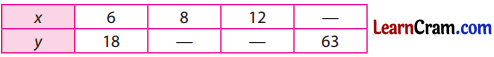Solution:
(i) Let us put with p, q and s in the blanks$$\frac{6}{18}=\frac{8}{p}$$
⇒ 6p = 8 × 18
⇒ p = $$\frac{8 \times 18}{6}$$
⇒ p = 8 × 3 = 24

$$\frac{6}{18}=\frac{12}{q}$$
⇒ 6q = 18 × 12
⇒ q = $$\frac{18 \times 12}{6}$$
⇒ q = 3 × 12 = 36

and $$\frac{6}{18}=\frac{s}{63}$$
⇒ 18s = 6 × 63
⇒ s = $$\frac{6 \times 63}{18}$$
⇒ s = 21.(ii)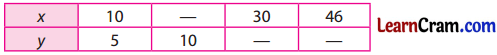Solution:
Let us put a, b and c in blanks of the given table.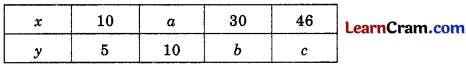$$\frac{10}{5}=\frac{a}{10}$$
⇒ 5 × a = 10 × 10
⇒ a = $$\frac{10 \times 10}{5}$$ = 20

$$\frac{10}{5}=\frac{30}{b}$$
⇒ 10 × b = 5 × 30
⇒ b = $$\frac{5 \times 30}{10}$$ = 15

and $$\frac{10}{5}=\frac{46}{c}$$
⇒ 10 × c = 5 × 46
⇒ c = $$\frac{5 \times 46}{10}$$
⇒ c = 23

(iii)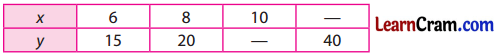Solution:
Let us fill the blanks with l and m in the given table.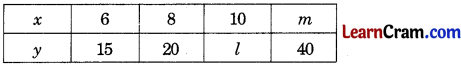∴ $$\frac{6}{15}=\frac{10}{l}$$
⇒ 6l = 10 × 15
⇒ l = $$\frac{10 \times 15}{6}$$ = 25

and $$\frac{6}{15}=\frac{m}{40}$$
⇒ 15 × m = 6 × 40
⇒ m = $$\frac{6 \times 40}{15}$$ = 16.Question 2.
Five bags of rice weigh 150 kg. How many such bags of rice will weigh 900 kg?
Solution:
Let the number of bags be x.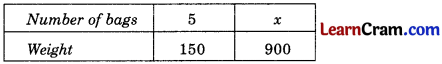As there is a direct variation,
$$\frac{5}{100}=\frac{x}{900}$$
⇒ 100 x = 5 × 900
⇒ x = $$\frac{5 \times 900}{100}$$ = 45 bags
Hence, the required bags are 45.

Question 3.
The cost of 3 kg of sugar is ₹ 54. What will be the cost of 15 kg of sugar?
Solution:
Let the required cost be ₹ x.As there is a direct variation,
$$\frac{3}{54}=\frac{15}{x}$$
⇒ 3x = 15
⇒ x = $$\frac{15 \times 54}{3}$$
⇒ x = 5 × 54 = 270.
Hence, the required cost is ₹ 270.

Question 4.
A motor boat covers 20 km in 4 hours. What distance will it cover in 7 hours (speed remaining the same)
Solution:
Let the distance covered be x km.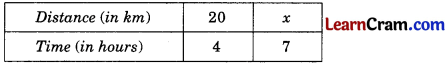As there is a direct variation,
∴ $$\frac{20}{4}=\frac{x}{7}$$
⇒ 4x = 20 × 7
⇒ x = $$\frac{20 \times 7}{4}$$
⇒ 4x = 5 × 7 = 35 km
Hence, the required distance = 35 km.Question 5.
A motor bike travels 210 km on 30 litres of petrol. How far would it travel on 7 litres of petrol?
Solution:
Let the distance travelled be x km.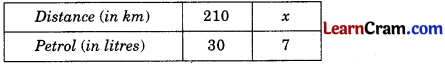As there is a direct variation,
∴ $$\frac{210}{30}=\frac{x}{7}$$
⇒ 30x = 210 × 7
⇒ x = $$\frac{210 \times 7}{30}$$
⇒ x = 7 × 7 = 49 km
Hence, the required distance is 49 km.

Question 6.
If 12 women can weave 15 m of cloth in a day, how many metres of cloth can be woven by 20 women in a day?
Solution:
Let the required length of the cloth be x m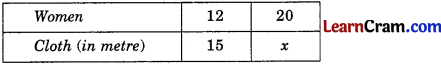As there is a direct variation,
∴ $$\frac{12}{15}=\frac{20}{x}$$
⇒ 12 × x = 15 × 20
⇒ x = $$\frac{15 \times 20}{12}$$
⇒ x = 25
Hence the length of the required cloth is 25 m.

Question 7.
If the weight of the 5 sheets of paper is 20 gram, how many sheets of the same paper would weigh 2.5 kg?
Solution:
Let the number of sheets required be x.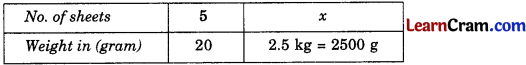As there is a direct variation,
∴ $$\frac{5}{20}=\frac{x}{2500}$$
⇒ 20 × x = 5 × 2500
⇒ x = $$\frac{5 \times 2500}{20}$$
⇒ x = 625
Hence the number of sheets required = 625.Question 8.
Reena types 600 words in 4 minutes. How much time will she take to type 3150 words?
Solution:
Let the time required be x minutes.As there is a direct variation,
∴ $$\frac{600}{4}=\frac{3150}{x}$$
⇒ 600 × x = 4 × 3150
⇒ x = $$\frac{4 \times 3150}{600}$$
⇒ x = 21
Hence, the required time is 21 minutes.

Question 9.
Rohan takes 14 steps in covering a distance of 2.8 m. What distance would he cover in 150 steps?
Solution:
Let the required distance be x m.As there is a direct variation,
∴ $$\frac{14}{2.8}=\frac{150}{x}$$
⇒ 14 × x = 2.8 × 150
⇒ x = $$\frac{2.8 \times 150}{14}$$
⇒ x = 30.
Hence, the required distance is 30 m.

Question 10.
A dealer finds that 48 refined oil cans (of 5 litres each) can be packed in 8 cartons of the same size. How many such cartons will be required to pack 216 cans?
Solution:
Let the number of cartons required be x.As there is a direct variation,
∴ $$\frac{48}{8}=\frac{216}{x}$$
⇒ 48 × x = 8 × 216
⇒ $$\frac{8 \times 216}{48}$$
⇒ x = 36.
Hence, the number of cartons required is 36.Question 11.
The total cost of 15 newspapers is ₹ 37.50. Find the cost of 25 newspapers.
Solution:
Let the required cost be ₹ x.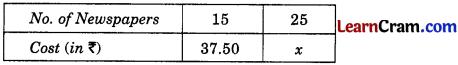As there is a direct variation,
∴ $$\frac{15}{37.50}=\frac{25}{x}$$
⇒ 15 × x = 25 × 37.50
⇒ $$\frac{25 \times 37.50}{15}$$
⇒ x = 62.50
Hence, the cost of 25 newspapers is ₹ 62.50.

Question 12.
A labourer gets ₹ 675 for 9 days work. How many days should he work to get ₹ 900?
Solution:
Let the number of days required be x.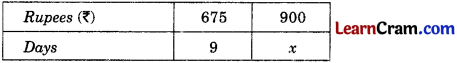∴ $$\frac{675}{9}=\frac{900}{x}$$
⇒ 675 × x = 9 × 900
⇒ x = $$\frac{9 \times 900}{675}$$
⇒ x = 12.
Hence, the number of days required is 12 days.

### DAV Class 8 Maths Chapter 4 Worksheet 1 Notes

Direct Variation:

• Let x be the number of members in a particular family and their monthly expenditure is ₹ y.
• If the number of the family members, i.e. x increases, their monthly expenditure, i.e. y also increases.
• On the other hand, if the number of members, i.e. x decreases, their monthly expenditure, i.e. y also decreases.
This is called the Direct Variation.

Inverse Variation:

Suppose, x men finish a work in y days. If the number of men increases, then the work will be finished in less than y days and if the number of men decreases, the days will be increased to finish the same work in time. So, we can say that x and y are inversely proportional to each other which is known as Inverse Variation.DIRECT VARIATION

Example 1.
Find the value of a and b in the following table, if x and y vary directly.

 x 3 a 18 y 5 35 b

Solution:
Given that x and y vary directly.
∴ $$\frac{3}{5}=\frac{a}{35}=\frac{18}{b}$$
⇒ $$\frac{3}{5}=\frac{a}{35}$$
⇒ 5a = 3 × 35
⇒ a = $$\frac{3 \times 35}{5}$$
⇒ a = 3 × 7 = 21
Now taking $$\frac{3}{5}=\frac{18}{b}$$
⇒ 3b = 5 × 18
⇒ b = $$\frac{5 \times 18}{3}$$
⇒ b = 5 × 6
∴ b = 30

Example 2.
In the following table, verify that x and y are directly proportional.Solution:
Here,
$$\frac{x}{y}=\frac{11}{22}=\frac{1}{2}$$
$$\frac{x}{y}=\frac{8}{16}=\frac{1}{2}$$
$$\frac{x}{y}=\frac{5}{10}=\frac{1}{2}$$
$$\frac{x}{y}=\frac{17}{34}=\frac{1}{2}$$
$$\frac{x}{y}=\frac{1}{2}$$ (k constant)
∴ x and y are directly proportional to each other.Example 3.
The cost of 5 metres of cloth is ₹ 210. Tabulate the cost of 2, 4, 10 and 13 metres of cloth of the same type.
Solution:
Let the length of cloth be x m and its cost be ₹ y.
Forming the table,As x and y are directly proportional.
∴ $$\frac{2}{y_1}=\frac{5}{210}$$
⇒ 5y1 = 2 × 210
⇒ y1 = $$\frac{2 \times 210}{5}$$
= 2 × 42 = ₹ 84
Similarly,
$$\frac{4}{y_2}=\frac{5}{210}$$
⇒ 5y2 = 4 × 210
⇒ y2 = $$\frac{4 \times 210}{5}$$
⇒ y2 = 4 × 42 = ₹ 168

$$\frac{10}{y_3}=\frac{5}{210}$$
⇒ 5y3 = 10 × 210
⇒ y3 = $$\frac{10 \times 210}{5}$$
⇒ y3 = ₹ 420

$$\frac{13}{y_4}=\frac{5}{210}$$
⇒ 5y4 = 13 × 210
⇒ y4 = $$\frac{13 \times 210}{5}$$
⇒ y4 = 13 × 42 = ₹ 546.Example 4.
If the weight of 10 sheets of thick paper is 50 gram, how many sheets of the same paper would weigh 2$$\frac{1}{2}$$ kg?
Solution:
Let the number of sheets required be x.

 Number of sheets 10 x Weight. of sheets (in gram) 50 2500

As there is a direct variation,
$$\frac{10}{50}=\frac{x}{2500}$$
⇒ 50 × x =10 × 2500
⇒ x = $$\frac{10 \times 2500}{50}$$
⇒ x = 500
Hence, the required number of sheets is 500.